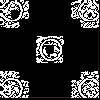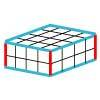#### You may also like### Doplication

We can arrange dots in a similar way to the 5 on a dice and they usually sit quite well into a rectangular shape. How many altogether in this 3 by 5? What happens for other sizes?### Round and Round the Circle

What happens if you join every second point on this circle? How about every third point? Try with different steps and see if you can predict what will happen.### Making Cuboids

Let's say you can only use two different lengths - 2 units and 4 units. Using just these 2 lengths as the edges how many different cuboids can you make?

# Round the Four Dice

##### Age 7 to 11Challenge Level
Begin by rolling the dice and making four different four-digit numbers. Then round each of them to the nearest multiple of 1000. Remember that sometimes you round up and sometimes you round down.

You could record your results in a table like this one:

 Numbers rolled 1st four-digit number Rounds to 2nd four-digit number Rounds to 3rd four-digit number Rounds to 4th four-digit number Rounds to 1, 2, 3 and 4 1324 1000 2314 2000 4312 4000 4123 4000